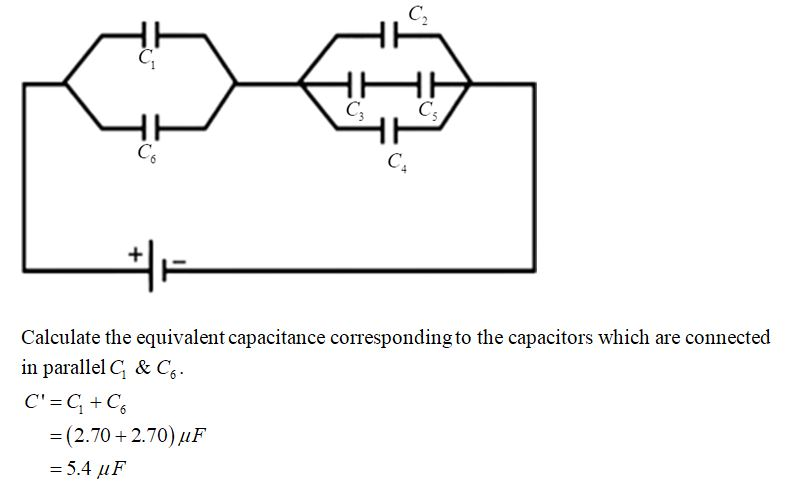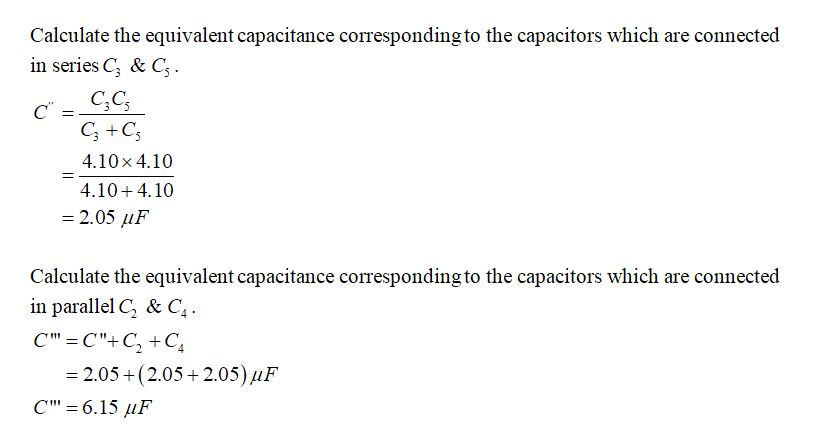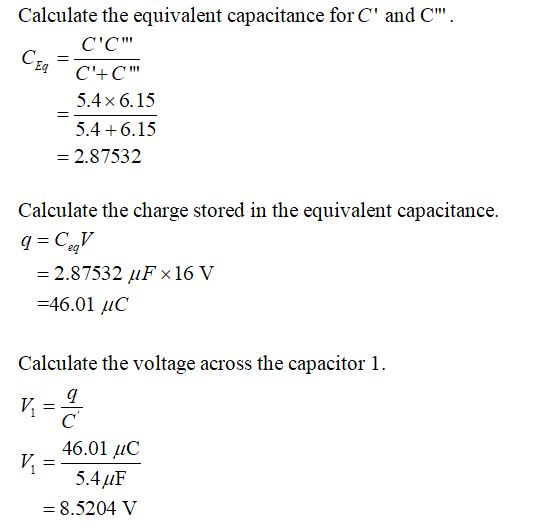Question
1 views

In the figure below, a 16.0 V battery is connected across capacitors of capacitances with the following values.

C1 = C6 = 2.70 μF
C3 = C5 = 4.10 μF
C2 = C4 = 2.05 μF

(d) What is q1 of capacitor 1?
______________C

(e) What is V2 of capacitor 2?
______________V

(f) What is q2 of capacitor 2?
_______________C
check_circle

Step 1

Draw the equivalent circuit for the given circuit diagram:Step 2Step 3...

### Want to see the full answer?

See Solution

#### Want to see this answer and more?

Solutions are written by subject experts who are available 24/7. Questions are typically answered within 1 hour.*

See Solution
*Response times may vary by subject and question.
Tagged in